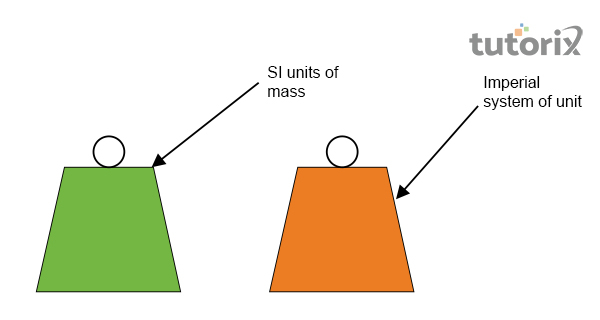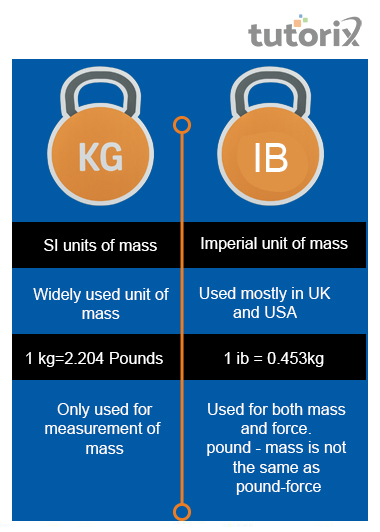# Difference Between Pound and Kilogram

## Introduction

In physics, to measure the physical quantity, different units are generally used. These units also involve some comparison amongst them. In simple diction, the measurement units can be defined by the magnitude of quantity.

That is used as a standard for measuring the properties that share some similarities. Based on this particular concept of physics, this tutorial will explain the differences that are found between two units of solid physician quantity measurement.

## What is Pound?Figure 1: Kilogram and pound

The pound has been considered one of the units that are used in order to measure the mass of any solid physical quantity. However, this unit shares a basic difference with the other unit of mass which is kg. In accordance with this fact, the pound is generally known as the core unit of measuring the mass that is based on the imperial system.

The other units of measurement of solid mass according to ti the imperial system are ounce and stone. Ib (Physicscatalyst, 2022) represents the abbreviation of this particular unit of mass. The main reason for this representation is that pound is usually associated with the Roman Libra. In addition, different varieties of this nit have been found in different units of measurement. The measurement of one pound is contemplated to be equivalent to the value of 0.45359237 Kg (Isoyama et al. 2022).

it has been seen that the altered value of the pound that is represented in kg is significant and difficult to know as the exact way it is. Therefore, the simplest version that is acknowledged generally is that the value of one pound is equal to 0.450 Kg

## What is Kilogram?

This particular measuring unit of the solid mass is generally represented as an abbreviation that is kg. This unit is actually acknowledged worldwide as the measuring unit of the SI system where the measurement of one kg is considered to be equivalent to the mass expressed through one litter of water (Davidson & Stock, 2021).

The Iridium-Platinum cylindrical figure of thus nit is IPK which is equal to 1 kg. International Bureau of Weights and Measures has stored this in a vault in France.

### Pounds to kilogram formula

In order to find the formula to convert the value of one pound into another unit that is kg is generally represented by a mathematical function. The mathematical formula for converting the value of one pound into the other unit kilogram is that the value of the pound needs to be multiplied by the determined value of 0.45359237 kg (Bbc, 2022).

In some cases, 0.454 multiplies the value that is represented in ib in order to convert the value into a kilogram. Based on this formula, for instance, if one physical quantity is measured to have 65 pounds, it can be represented by 29.51 kilograms.

### The formula for the kilogram to pound

The value represented in a kilogram can be converted into a pound based on a specific mathematical formula. The measure of one kilogramme is considered to be equivalent to 2.2 lb. Therefore, it can be stated that the value that is represented in kg can be converted into pounds by multiplying the provided value by 2.2.

### Differences between Pound and KilogramFigure 2: Differences between kilogram and pound

Two units that are usually used in order to get the value of a solid mss shares some differences. One of the main differences that are found to be shared between these two units of measurement is that the kilogram is acknowledged as the SI unit. On the contrary, the pound is known to be the imperial unit of mass. The short form or the abbreviation of kilogram is kg. In contradiction, the abbreviation through which pound can be represented is Ib as this is considered to be descended from Roman libra.

The unit of measurement of solid mss that is commonly used is kilogram, whereas, the unit of the pound is generally used in the United Kingdom and the United SAtes of America. On one hand, the value that is considered equivalent to one kilogram is 2.204 pounds (Askanydifference, 2022). On the other hand, the value of one pound is represented by 0.453Kg. The core difference is that the kilogram is used for getting the measured quantity of mass of any solid object, whereas, the pound represents both mass and force.

## Conclusion

The main units that are mostly used for the measurement of solid mass are pounds and kilograms. The imperial system of the measurement of a solid physical quantity is called the pound. On the other hand, in the international system of the unit, the SI unit of measurement of solid mass is called kilogram. The core difference lies between the two units of mass is that a kilogram refers to the mass of the physical unit, on the contrary, a pound refers to both mass and force at the same time

### FAQs

Q1. Defines the types of unit?

According to physics, there are two types of nits are available for the measurement of the physical quantities. One is the fundamental unit that is referred to as the base or fundamental quantities. On the other hand, the derived unit is referred to a combined representation of base units.

Q2. Why pound is used instead of Kg?

One kilogram is equivalent to the approximate quantity of 2.2 pounds. In accordance with this statement, it can be found that a kilo can be considered 2.2 times heavier in comparison to a pound.

Q3. What will be the value of 10 pounds of apple if measured in kg?

The provided measurement of the apple is 10 pounds. It has been known to all that one pound is equivalent to 0.450 kg. Therefore, 10 pounds of apple will be equivalent to $\mathrm{10*0.450\:kg}$. Thus, the value of 10 pounds of apple will be 4.5kg.